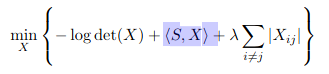# Absolute sum of non-diagonal elements

I have a minimization objective like this:In my code I am trying to represent it as:

``````   cvx_begin
variable X(size(A));
idx = eye(size(A));
Y = (1-idx).*X;
minimize ( -log_det(X) + S * X + lambda * Y );
cvx_end
``````

I am getting this error:

Your objective function is not a scalar.

A is a random matrix the same size as S.
Can you all please suggest what I am doing wrong? Thanks!

I suggest you read whatever paper or book you are getting this from more carefully and try to understand the material in it. Obviously, a non-scalar matrix objective function makes no sense, which would be apparent if you thought about it.

`<S,X>` means `trace(S'*X)`, which is an affine function which can be directly entered into CVX. It also happens to equal `sum(sum(S.*X))`, which could be alternatively used.

`S*X` is a matrix, and therefore, per the rules of MATLAB, so is the objective function. Hence the CVX error ,message.

I don’t believe `Y` is correct. I believe
`Y = trace((1-idx)*abs(X))`
or
`Y = sum(sum((1-idx).*abs(X)))` would do the trick.

Actually it’s an assignment question.

I defined X as:

`variable X(size(A));`

Well, it’s better for you to understand your assignments, not blindly do things which don’t make any sense. Please ask your Prof to give me an A.